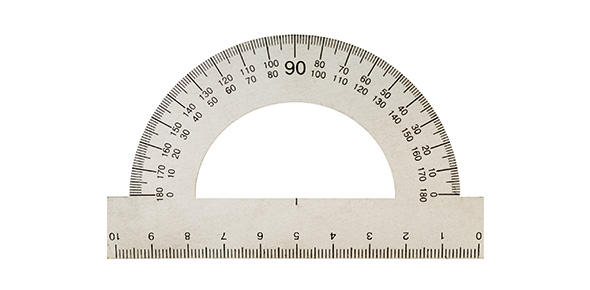# 1-2. Significant Figures And Scientific Notation

10 Questions | Total Attempts: 727SettingsScientific notation is the way that scientists easily handle very large numbers or very small numbers and very helpful when it comes to significant numbers. Identifying significant number can be confusing if one does not understand the rules on how to identify them. Take the quiz and test your understanding of both concepts.

• 1.
How many significant figures are in the following value: 351.0 cm
• 2.
Bob measured the width of a cardboard box to be 640 cm. As written, which of the following digits in his measurement is/are significant? Check all that apply.
• A.

6

• B.

4

• C.

0

• 3.
Sally found that the mass of an unknown solid is 0.5200 g. True or False: The rightmost zero in 0.5200 g is a significant digit.
• A.

True

• B.

False

• 4.
2.55 mL + 45.723 mL + 0.30 mL = _____. (The correct answer will have the correct number of significant figures.)
• A.

48.573 mL

• B.

48.57 mL

• C.

48.6 mL

• D.

49 mL

• 5.
4.56 km * 35.6 km = _____ km. (Note: the correct answer will have the correct number of significant figures.)
• 6.
How many significant figures are in the answer for the following calculation?:
• A.

2

• B.

3

• C.

4

• D.

5

• 7.
Vance wants to write the number 5670000 in scientific notation. Fill in the blank:
• 8.
Express the number 0.000466 in scientific notation by filling in the exponent:
• 9.
Express the following number in expanded form:
• A.

78,600

• B.

7,860

• C.

768

• D.

76.8

• 10.
Express the following number in expanded form:
• A.

0.000002563

• B.

0.00002563

• C.

2,563

• D.

256,300

Related TopicsBack to top